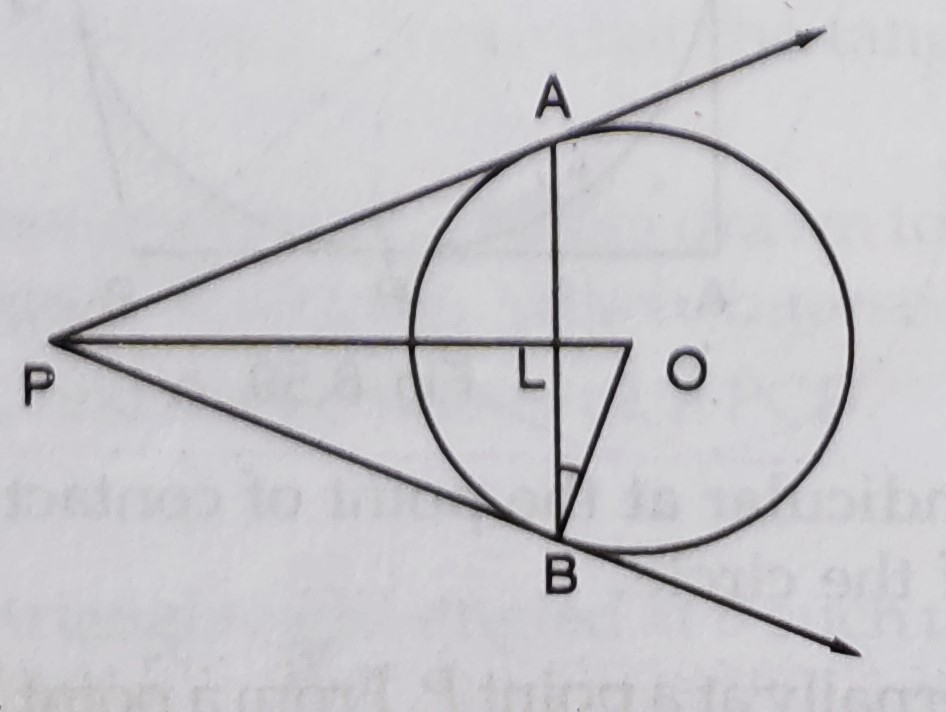"
">

# In the figure, $A B$ is a chord of length $16 \mathrm{~cm}$ of a circle of radius $10 \mathrm{~cm}$. The tangents at $A$ and $B$ intersect at a point $P$. Find the length of $P A$."

Given:

In the figure, $A B$ is a chord of length $16 \mathrm{~cm}$ of a circle of radius $10 \mathrm{~cm}$. The tangents at $A$ and $B$ intersect at a point $P$.

To do:

We have to find the length of $P A$.

Solution:

Join $OA$.

$OA = 10\ cm$

$OL$ is perpendicular to $AB$.

$AL=LB= \frac{16}{2}=8\ cm$  (AL and LB are the radii of the circle)

In right angled triangle $OLA$,

By Pythagoras theorem,

$OA^2=OL^2 +LA^2$

$OL^2=OA^2-LA^2$

$OL^2=(10)^2-8^2$

$=100-64$

$=36$

$\Rightarrow OL=6\ cm$

$\tan\ AOL=\frac{AL}{OL}$

$=\frac{8}{6}$

$=\frac{4}{3}$

From $\triangle OAP$,

$\tan\ AOL=\frac{PA}{OA}$

$\frac{4}{3}=\frac{PA}{10}$

$PA=\frac{10\times4}{3}$

$PA=\frac{40}{3}\ cm$

The length of PA is $\frac{40}{3}\ cm$.

Updated on: 10-Oct-2022

59 Views